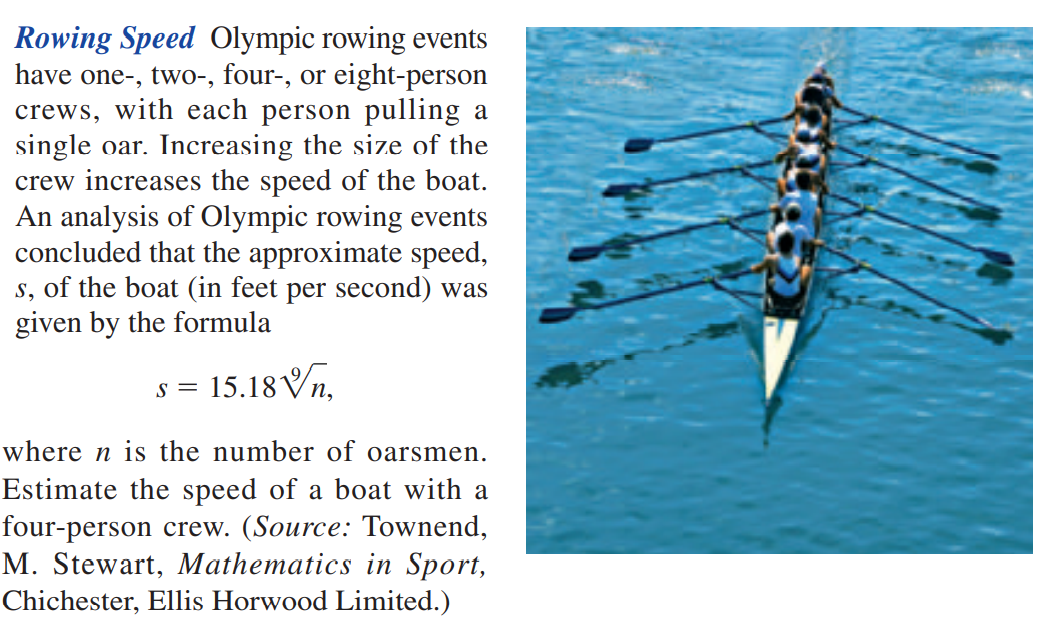### Still have math questions?

Algebra
QuestionRowing Speed Olympic rowing events have one- , two-, four-, or eight-person crews, with each person pulling a single oar. Increasing the size of the crew increases the speed of the boat. An analysis of Olympic rowing events concluded that the approximate speed,

$$s$$ , of the boat (in feet per second) was given by the formula

$$s = 15.18 \sqrt[ 9 ] { n }$$ , where $$n$$ is the number of oarsmen. Estimate the speed of a boat with a four-person crew. (Source: Townend, M. Stewart, Mathematics in Sport, Chichester, Ellis Horwood Limited.)